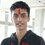# Finding $\sum \frac{1}{1+n^2}$

How do we prove that the sum $S=\sum_{n=0}^{\infty} \dfrac{1}{1+n^2}$ converges to $\dfrac{\pi+1}{2}+\dfrac{\pi}{e^{2\pi}-1}$?Note by Pratik Shastri
6 years, 8 months ago

This discussion board is a place to discuss our Daily Challenges and the math and science related to those challenges. Explanations are more than just a solution — they should explain the steps and thinking strategies that you used to obtain the solution. Comments should further the discussion of math and science.

When posting on Brilliant:

• Use the emojis to react to an explanation, whether you're congratulating a job well done , or just really confused .
• Ask specific questions about the challenge or the steps in somebody's explanation. Well-posed questions can add a lot to the discussion, but posting "I don't understand!" doesn't help anyone.
• Try to contribute something new to the discussion, whether it is an extension, generalization or other idea related to the challenge.
• Stay on topic — we're all here to learn more about math and science, not to hear about your favorite get-rich-quick scheme or current world events.

MarkdownAppears as
*italics* or _italics_ italics
**bold** or __bold__ bold
- bulleted- list
• bulleted
• list
1. numbered2. list
1. numbered
2. list
Note: you must add a full line of space before and after lists for them to show up correctly
paragraph 1paragraph 2

paragraph 1

paragraph 2

[example link](https://brilliant.org)example link
> This is a quote
This is a quote
    # I indented these lines
# 4 spaces, and now they show
# up as a code block.

print "hello world"
# I indented these lines
# 4 spaces, and now they show
# up as a code block.

print "hello world"
MathAppears as
Remember to wrap math in $$ ... $$ or $ ... $ to ensure proper formatting.
2 \times 3 $2 \times 3$
2^{34} $2^{34}$
a_{i-1} $a_{i-1}$
\frac{2}{3} $\frac{2}{3}$
\sqrt{2} $\sqrt{2}$
\sum_{i=1}^3 $\sum_{i=1}^3$
\sin \theta $\sin \theta$
\boxed{123} $\boxed{123}$

## Comments

Sort by:

Top Newest

Euler while solving basel problem considered the function $sin(x)$ as an infinte product as :

$sin(x)=x\displaystyle \prod _{ n=1 }^{ \infty }{ (1-\dfrac { { x }^{ 2 } }{ { (n\pi ) }^{ 2 } } ) }$

Taking $ln()$ both sides we get:

$ln(sin(x))=ln(x)+\displaystyle \sum _{ n=1 }^{ \infty }{ ln(1-\dfrac { { x }^{ 2 } }{ ({ n\pi })^{ 2 } } ) }$

Differentiating both sides with respect to $x$ we get :

$cot(x)=\dfrac { 1 }{ x } +\displaystyle \sum _{ n=1 }^{ \infty }{ \dfrac { 2x }{ { (n\pi ) }^{ 2 } } \dfrac { 1 }{ (\dfrac { { x }^{ 2 } }{ { (n\pi ) }^{ 2 } } -1) } }$

$\Rightarrow cot(x)=\dfrac { 1 }{ x } +\displaystyle \sum _{ n=1 }^{ \infty }{ \dfrac { 2x }{ ({ x }^{ 2 }-{ (n\pi ) }^{ 2 }) } }$

Putting $\pi x$ instead of $x$ we get :

$cot(\pi x)=\dfrac { 1 }{ \pi x } +\dfrac { 1 }{ \pi } \displaystyle \sum _{ n=1 }^{ \infty }{ \frac { 2x }{ ({ x }^{ 2 }-{ n }^{ 2 }) } }$

Multiplying both sides with $\pi x$ we get :

$\pi xcot(\pi x)=1+{ 2x }^{ 2 } \displaystyle \sum _{ n=1 }^{ \infty }{ \frac { 1 }{ ({ x }^{ 2 }-{ n }^{ 2 }) } }$

Also we know that $icot(ix)=coth(x)$

Putting $ix$ in place of $x$ we get :

$\pi xcoth(\pi x)=1+2{ x }^{ 2 } \displaystyle \sum _{ n=1 }^{ \infty }{ \frac { 1 }{ { x }^{ 2 }+{ n }^{ 2 } } }$

Put $x=1$ to get :

$\pi coth(\pi )=1+2\displaystyle \sum _{ n=1 }^{ \infty }{ \frac { 1 }{ n^{ 2 }+{ 1 } } }$

$=2\displaystyle \sum _{ n=0 }^{ \infty }{ \frac { 1 }{ n^{ 2 }+{ 1 } } } -1$

$\Rightarrow \displaystyle \sum _{ n=0 }^{ \infty }{ \frac { 1 }{ n^{ 2 }+{ 1 } } } =\frac { \pi coth(\pi )+1 }{ 2 }$

$\Rightarrow \displaystyle \sum_{n=0}^{\infty}{\frac{1}{n^{2}+1}}=\frac{\pi+1}{2}+\frac{\pi}{e^{2\pi}-1}$

- 6 years, 8 months ago

Log in to reply

That's some identity, isn't it? Nice solution by the way!

- 6 years, 8 months ago

Log in to reply

From where you found the question.

- 6 years, 8 months ago

Log in to reply

I didn't find it from anywhere. I was fiddling around with infinite series and I typed this into wolfram alpha.

- 6 years, 8 months ago

Log in to reply

From where you get these type of identities.

- 6 years, 8 months ago

Log in to reply

Can you please prove that $icot(ix) = coth(x)$

- 6 years, 5 months ago

Log in to reply

Easy, we know that :

$cos(ix) = \frac{{e}^{x}+{e}^{-x}}{2}$

Also $sin(ix) = \frac{{e}^{x}-{e}^{x}}{2i}$

Dividing then we get :

$cot(ix) = (\frac{{e}^{x}+{e}^{-x}}{{e}^{x}-{e}^{-x}})i$

Hence finally we have :

$cot(ix)=icoth(x)$

- 6 years, 5 months ago

Log in to reply

If somebody wants to overkill it, Then

$S = \sum_{n=0}^{\infty} \frac{1}{1+n^2} \\ \\ = \frac{1}{2i} \sum_{n=0}^{\infty} \left( \frac{1}{n-i} - \frac{1}{n+i} \right)$ Now , using the unique property of digamma function that is it satisfies, $\psi(x+1)-\psi(x)=\frac{1}{x}$

We get that , sum is equivalent to, $1+ \frac{\psi(1+i) - \psi(1-i)}{2i}$ Now, again using reflection formula, this can be easily calculated and it equals,

$\frac{1}{2} + \frac{\pi}{2} \coth \pi = \frac{\pi+1}{2} + \frac{\pi}{e^{2\pi}-1}$

- 6 years, 8 months ago

Log in to reply

Are you 16 years old and know digamma function? Wow!

- 6 years, 5 months ago

Log in to reply

- 6 years, 8 months ago

Log in to reply

×

Problem Loading...

Note Loading...

Set Loading...